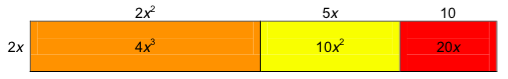## Multiplying a Polynomial by a Monomial

### Learning Outcomes

• Multiply a polynomial by a monomial

In the exponents section, we practiced multiplying monomials together, like we did with this expression: $24{x}^{8}2{x}^{5}$. The only thing different between that section and this one is that we called it simplifying, and now we are calling it polynomial multiplication.  Remember that simplifying a mathematical expression means performing as many operations as we can until there are no more to perform, including multiplication.  In this section we will show examples of how to multiply more than just monomials.  We will multiply monomials with binomials and trinomials. We will also learn some techniques for multiplying two binomials together.

### Example

Multiply. $-9x^{3}\cdot 3x^{2}$

That’s it! When multiplying monomials, multiply the coefficients together, and then multiply the variables together. Remember, if two variables have the same base, follow the rules of exponents, like this:

$\displaystyle 5{{a}^{4}}\cdot 7{{a}^{6}}=35{{a}^{10}}$

The following video provides more examples of multiplying monomials with different exponents.

## Find the product of polynomials and monomials

Previously, you learned to use the Distributive Property to simplify expressions such as $2\left(x - 3\right)$. You multiplied both terms in the parentheses, $x\text{ and }3$, by $2$, to get $2x - 6$. With this chapter’s new vocabulary, you can say you were multiplying a binomial, $x - 3$, by a monomial, $2$. Multiplying a binomial by a monomial is nothing new for you!  The distributive property can be used to multiply a monomial and a binomial. Just remember that the monomial must be multiplied by each term in the binomial.

### example

Multiply: $3\left(x+7\right)$

Solution

 $3\left(x+7\right)$ Distribute.$3\cdot x+3\cdot 7$ Simplify. $3x+21$

### example

Multiply: $x\left(x - 8\right)$

### example

Multiply: $10x\left(4x+y\right)$

### try it

In the next example, you will see how to multiply a second degree monomial with a binomial.  Note the use of exponent rules.

### Example

Simplify. $5x^2\left(4x^{2}+3x\right)$

Now let’s add another layer by multiplying a monomial by a trinomial. Multiplying a monomial by a trinomial works in much the same way as multiplying a monomial by a binomial.  Consider the expression $2x\left(2x^{2}+5x+10\right)$.

This expression can be modeled with a sketch like the one below.The only difference between this example and the previous one is there is one more term to distribute the monomial to.

$\begin{array}{c}2x\left(2x^{2}+5x+10\right)=2x\left(2x^{2}\right)+2x\left(5x\right)=2x\left(10\right)\\=4x^{3}+10x^{2}+20x\end{array}$

You will always need to pay attention to negative signs when you are multiplying. Watch what happens to the sign on the terms in the trinomial when it is multiplied by a negative monomial in the next example.

### Example

Simplify. $-7x\left(2x^{2}-5x+1\right)$

### example

Multiply: $-2x\left(5{x}^{2}+7x - 3\right)$

### example

Multiply: $4{y}^{3}\left({y}^{2}-8y+1\right)$

### try it

Now we will have the monomial as the second factor.

### example

Multiply: $\left(x+3\right)p$

### try it

In the following video we show more examples of how to multiply monomials with other polynomials.

## Contribute!

Did you have an idea for improving this content? We’d love your input.